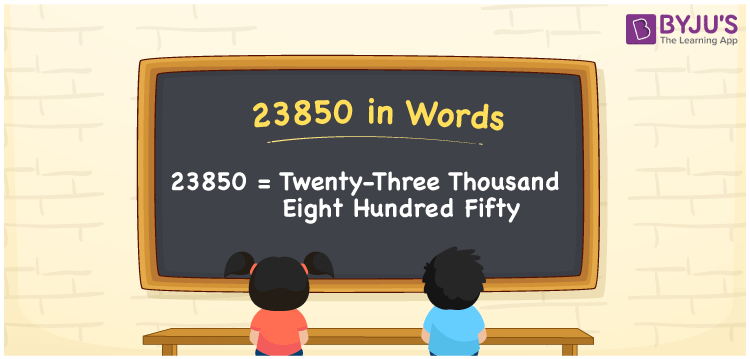# 23850 in Words

The number 23850 in words is Twenty-three Thousand Eight Hundred Fifty. If you bought the clothes for Rs. 23850, it can be written as “I bought the clothes for Rs. Twenty-three thousand eight hundred fifty. We know that the number 23850 is a cardinal number and it has five digits. Here is a detailed explanation of writing the number 23850 in words using the place value system.

 23850 in Words: Twenty-three Thousand Eight Hundred Fifty. Twenty-three Thousand Eight Hundred Fifty in Numerical Form: 23850.

## 23850 in English Words## How to Write 23850 in Words?

Learn the place values of the number 23850 using the below table.

 Ten-thousands Thousands Hundreds Tens Ones 2 3 8 5 0

The expanded form of 23850 is as follows:

= 2 × Ten thousand + 3 × Thousand + 8 × Hundred + 5 × Ten + 0 × One

= 2 × 10000 + 3 × 1000 + 8 × 100 + 5 × 10 + 0 × One

= 20000 + 3000 + 800 + 50

= 23850

= twenty-three thousand eight hundred fifty

Hence, 23850 in words is twenty-three thousand eight hundred fifty.

23850 in words – Twenty-three thousand eight hundred fifty

Is 23850 an odd number? – No

Is 23850 an even number? – Yes

Is 23850 a perfect square number? – No

Is 23850 a perfect cube number? – No

Is 23850 a prime number? – No

Is 23850 a composite number? – Yes

## Frequently Asked Questions on 23850 in Words

Q1

### Write 23850 in words.

23850 in words is twenty-three thousand eight hundred fifty.

Q2

### Simplify 23000 + 850, and express it in words.

Simplifying 23000 + 850, we get 23850. Hence, 23850 in words is twenty-three thousand eight hundred fifty.

Q3

### Is 23850 an even number?

Yes, 23850 is an even number.